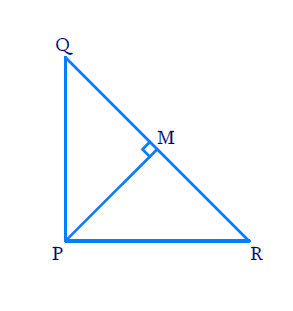# Ex.6.5 Q2 Triangles Solution - NCERT Maths Class 10

Go back to  'Ex.6.5'

## Question

$${PQR}$$ is a triangle right angled at $$P$$ and $$M$$ is a point on $$QR$$ such that $$PM \bot QR$$. Show that $${{(\text{PM})}^{2}}$$ = $${QM. MR}$$

Diagram## Text Solution

Reasoning:

As we know if a perpendicular is drawn from the vertex of the right angle of a right triangle to the hypotenuse then triangles on both sides of the perpendicular are similar to the whole triangle and to each other.

Steps:

In $$\,\Delta PQR\,;\,\angle QPR={{90}^{0}}$$ and $$PM\bot QR$$

In $$\,\Delta PQR\,$$ and $$\,\Delta MQP\,$$

$$\angle Q P R=\angle Q M P=90^{\circ}$$

$$\angle P Q R=\angle M Q P$$ (Common Angles)

\begin{align} {\Rightarrow} \quad \Delta P Q R \sim \Delta M Q P\end{align} (AA Criterion)......-(1)

In $$\Delta PQR$$ and $$\Delta MPR$$

$$\angle QMP=\angle PMR={{90}^{0}}$$

$$PRQ= RPM$$ (Common Angle)

\begin{align}\Rightarrow\quad \,\Delta \,PQR&\sim{\ }\Delta \,MPR\end{align}  (AA Criterion).........(2)

From (1) and (2)

$$\Delta MQP\sim{\ }\Delta MPR$$

\begin{align}\frac{P M}{M R}&=\frac{Q M}{P M} \text{[Comparing sides opposite to equal angles]}\\&{\Rightarrow\quad P M^{2}=Q M \cdot M R}\end{align}

Learn from the best math teachers and top your exams

• Live one on one classroom and doubt clearing
• Practice worksheets in and after class for conceptual clarity
• Personalized curriculum to keep up with school